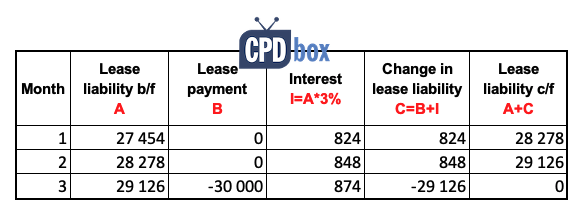## Question

We took the lease contract to rent a building for 6 years with total cost of CU 60 000, of which:

• CU 30 000 is paid up front before we moved in; and
• CU 30 000 is paid at the beginning of the 4th year.

How should we account for the above?

### Initial recognition and measurement

Under IFRS 16, you shall recognize the right-of-use (ROU) asset and the lease liability.

#### Lease liability:

The lease liability is calculated as the present value of the lease payments not paid at the commencement date discounted with the interest rate implicit in the lease (IRR), or the incremental borrowing rate if IRR cannot be determined.

So, in this case, there is only one payment not paid: CU 30 000 to be paid at the beginning of the 4th year.

And, in most cases, it will be difficult and impractical to determine the IRR, so we take the incremental borrowing rate. More on that topic here. For the purpose of this example, let’s say we take the similar borrowing rate of 3%.

Special For You! Have you already checked out the  IFRS Kit ? It’s a full IFRS learning package with more than 40 hours of private video tutorials, more than 140 IFRS case studies solved in Excel, more than 180 pages of handouts and many bonuses included. If you take action today and subscribe to the IFRS Kit, you’ll get it at discount! Click here to check it out!

You can discount CU 30 000 with 3% with one simple formula. Just multiply CU 30 000 with the discount factor for 3% and 3 years. You can calculate the discount factor with the following formula:Just please bear in mind that you are discounting for 3 years, not 4 – the payment was to be done at the beginning, not at the end of the year 4, so in fact it is in 3 years time.

The present value is then CU 27 454 which is equal to the lease liability in this particular case.

#### ROU asset:

Here, the ROU asset is calculated as the sum of:

• The lease payments made at of before the lease commencement date. Here, this is equal the first payment of CU 30 000 made up front before you moved in.
• The amount of the initial measurement of the lease liability – see above, calculated at CU 27 454.

The ROU asset is then CU 57 454, and your initial journal entry is:

• Debit ROU asset: CU 57 454

• Credit Lease liability: CU 27 454

• Credit Bank account (cash paid): CU 30 000

### Subsequent measurement

#### Lease liability:

You would need to accrue interest each year, even if no lease payment is made, until you have some lease liability left.

To do that, the easiest way is to set up a table:As you can see, the lease liability after the second payment becomes zero, which is OK because there are no payments left to make from the year 4 onwards.

Thus in the years 4, 5 and 6, you will still show ROU asset as the lease term is ongoing, but no lease liability since there will be none.

Journal entries:

At the end of year 1, year 2, and year 3 you just accrue the interest:

• Debit Interest expense in P/L: CU 824 (year 1) or CU 848 (year 2) or CU 874 (year 3)

• Credit Lease liability: CU 824 (year 1) or CU 848 (year 2) or CU 874 (year 3)

At the beginning of the year 4, the lease liability becomes CU 27 454 (initial amount) plus CU 824 (interest in year 1) plus CU 848 (interest in year 2) plus CU 874 (interest in year 3), which is – not surprisingly – CU 30 000.

You pay the second payment, then:

• Debit Lease liability: CU 30 000

• Credit Cash: CU 30 000

Of course, do NOT forget to depreciate the ROU asset, maybe on a straight-line basis over the lease term of 6 years. Thus at the end of each year, the entry will be:

• Debit Depreciation in P/L: CU 9 576 (which is ROU asset of CU 57 454 divided by 6)

• Credit ROU asset: CU 9 576

So, if you have any questions, feel free to ask below in the comments.